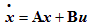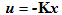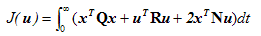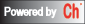The state-space method is the modern approach for control system design and analysis. The controllability and observability are important structural properties of a control system. The controllability and observability analysis below can be used to check whether the system is controllable or observable. The controllability and observability staircase are useful when the system has uncontrollable or unobservable states. The function grammian computes the controllability and observability grammians of a state-space model.

Pole placement design is one of the attractive feature of the state-space design method. It allows us to place closed-loop poles to locations that will correspond to desired dynamic response. Given the state equationand a desired closed-loop pole vector p, pole placement computes a gain matrix K according to (A, B) and p such that the full-state feedbackplaces the closed-loop poles at the desired locations.

The purpose of LQG design is to find an optimal gain matrix K such that the state-feedback lawminimizes the cost function J(u).Function
`    `
Description
Controllability analysis Check whether a system is controllable.
Controllability staircase Compute the controllability staircase form.
Grammian Compute the controllability and observability grammians of a state-space model.
LQE design Kalman estimator design for continuous-time systems.
LQG design Design optimal linear quadratic state-feedback regulator for continuous-time plant.
Lyapunov equation solvers Solve Lyapunov equation.
Observability analysis Check whether a system is observable.
Observability staircase Compute observability staircase form.
Pole placement Compute the feedback gain matrix k such that the closed loop poles are at the desired locations.